# Communication channel

(diff) ← Older revision | Latest revision (diff) | Newer revision → (diff)

One of the main constituents of systems for the transmission of information considered in the theory of information transmission. It is used in the mathematical description of an actual communication channel, that is, the complex of technical apparatus enabling one to transmit information from a transmitter to a receiver over physical communication lines and media in which signals are propagated from the transmitter to the receiver.

A communication channel with one transmitter and one receiver used for the transmission of information in one direction (from the transmitter to the receiver), is defined by a pair of measurable spaces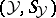,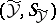(spaces of signals transmitted by the transmitter and received by the receiver, respectively), a transition function,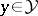,, which is measurable with respect to the-algebra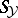for fixedand is a probability measure onfor each fixed(the function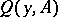gives the conditional probability distribution of the signal received by the receiver under the condition that the transmitter has transmitted the signal), and a subsetof the space of all probability measures on(defines a restriction on the distribution of the signal transmitted by the transmitter). Two random variablesand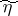defined on some probability space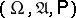are connected by the channel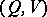if they take values inand, respectively, if for any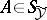the conditional probability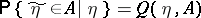with probability 1, and if the distribution ofbelongs to.

In applications one often encounters communication channels for whichandare spaces of measurable functions defined on an intervaland taking values, respectively, in measurable spacesand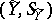with the-algebra generated by the measurable cylindrical sets. In this case one writes,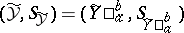and talks about a continuous-time communication channel on the interval, for whichandare random processes with values in the spaces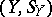and, respectively; the quantitiesand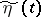are then regarded as the transmitted and received signals at the time. Similarly, in the discrete-time case, the input and output signals of the communication channel are given by random vectors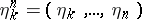andthe components of which take values in the measurable spacesand, respectively; here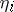and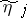are to be regarded as the transmitted and received signals at time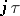, where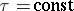is the time interval between two successive transmissions of signals over the communication channel.

Often continuous- or discrete-time communication channels are also considered on a semi-infinite interval or an interval which is infinite on both sides. For example, by a continuous-time communication channelon the semi-infinite intervalone means an ordinary family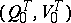of communication channels as described above, defined on all finite intervals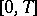and satisfying certain compatibility conditions. In this case each communication channelis called thesegment of the communication channel. One of the possible variants of the compatibility conditions can be stated as follows. Letbe an arbitrary set in, letbe an arbitrary function in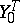and let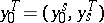for some,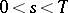, where,. Then the compatibility condition imposed on the transition functions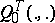is that for any, any,, anyand functions,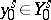,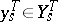,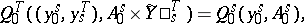whereis the cylindrical set in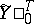generated by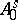in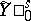. The restrictions,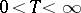, imposed on the probability measures inare also required to satisfy certain compatibility conditions.

The notion of a continuous-time communication channel on the infinite intervalis defined similarly. The compatibility conditions, necessary for the definition of such a channel, are usually formulated specifically for each type of channel. In some concrete situations, the notion of a communication channel onor on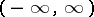is introduced directly (that is, without considering finite segments of these channels) by means of an explicit description of the processes performed on the input signal of the communication channel upon receipt of the output signal (see, for example, Gaussian channel; Additive noise). Everything said concerning a continuous-time communication channelon intervals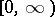orholds also for the analogous discrete-time communication channels.

Communication channels are divided into various classes depending on the types of the conditional distributionand of the restriction(see, for example, Memoryless channel; Channel with a finite memory; Channel with a finite number of states; Gaussian channel; Symmetric channel). A fundamental characteristic of a communication channel is the transmission rate of a channel (capacity), which characterizes the maximum possible transmission rate of information (cf. Information, transmission rate of) over this channel.

Various generalizations of communication channels, as defined above, are possible; these correspond to more general and complex systems of information transmission (see, for example, Channel with feedback; Channel with multiple directions).

How to Cite This Entry:
Communication channel. Encyclopedia of Mathematics. URL: http://encyclopediaofmath.org/index.php?title=Communication_channel&oldid=19102
This article was adapted from an original article by R.L. DobrushinV.V. Prelov (originator), which appeared in Encyclopedia of Mathematics - ISBN 1402006098. See original article